# ISEE Lower Level Quantitative : Quadrilaterals

## Example Questions

### Example Question #51 : Quadrilaterals

What area of the rectangle is filled in?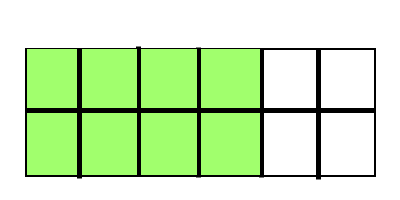Possible Answers: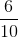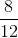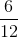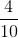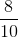Correct answer:Explanation:

Area is the amount of space within the perimeter of a two-dimensional shape. The rectangle is split intopieces andof those pieces are filled in.of the area of this rectangle is filled in.

### Example Question #4 : Find Area By Partitioning Shapes Into Equal Parts: Ccss.Math.Content.3.G.A.2

What area of the rectangle is filled in?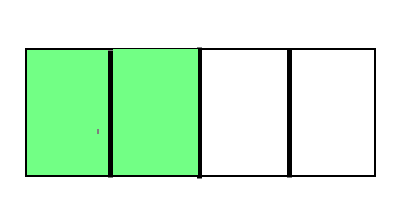Possible Answers: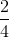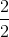Correct answer:Explanation:

Area is the amount of space within the perimeter of a two-dimensional shape. The rectangle is split intopieces andof those pieces are filled in.of the area of this rectangle is filled in.

### Example Question #31 : Geometry

What area of the rectangle is filled in?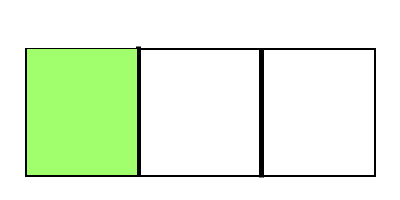Possible Answers:Correct answer:Explanation:

Area is the amount of space within the perimeter of a two-dimensional shape. The rectangle is split intopieces andof those pieces is filled in.of the area of this rectangle is filled in.

### Example Question #51 : Quadrilaterals

What area of the rectangle is filled in?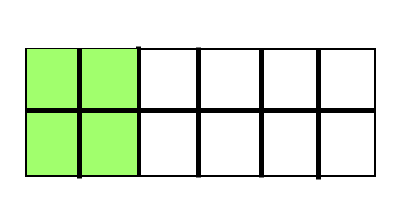Possible Answers: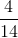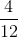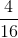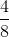Correct answer:Explanation:

Area is the amount of space within the perimeter of a two-dimensional shape. The rectangle is split intopieces andof those pieces are filled in.of the area of this rectangle is filled in.

### Example Question #5 : Find Area By Partitioning Shapes Into Equal Parts: Ccss.Math.Content.3.G.A.2

What area of the rectangle is filled in?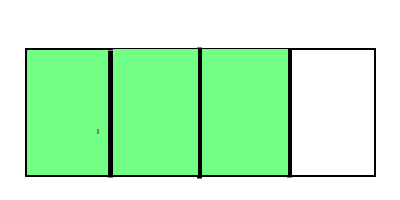Possible Answers: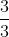Correct answer:Explanation:

Area is the amount of space within the perimeter of a two-dimensional shape. The rectangle is split intopieces andof those pieces are filled in.of the area of this rectangle is filled in.

### Example Question #32 : Geometry

What area of the rectangle is filled in?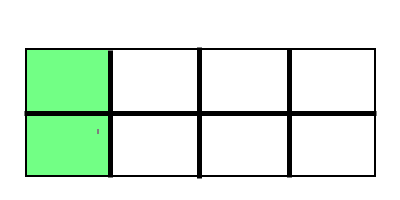Possible Answers: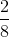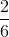Correct answer:Explanation:

Area is the amount of space within the perimeter of a two-dimensional shape. The rectangle is split intopieces andof those pieces are filled in.of the area of this rectangle is filled in.

### Example Question #31 : Geometry

What area of the rectangle is filled in?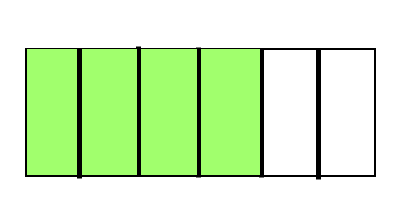Possible Answers: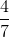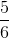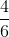Correct answer:Explanation:

Area is the amount of space within the perimeter of a two-dimensional shape. The rectangle is split intopieces andof those pieces are filled in.of the area of this rectangle is filled in.

### Example Question #11 : Find Area By Partitioning Shapes Into Equal Parts: Ccss.Math.Content.3.G.A.2

What area of the rectangle is filled in?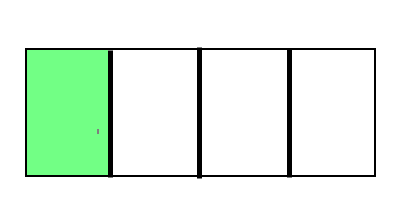Possible Answers: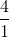Correct answer:Explanation:

Area is the amount of space within the perimeter of a two-dimensional shape. The rectangle is split intopieces andof those pieces is filled in.of the area of this rectangle is filled in.

### Example Question #1 : Geometric Measurement: Understanding Concepts Of Area And Relating Area To Multiplication And To Addition

How many square units make up the area of the shape below?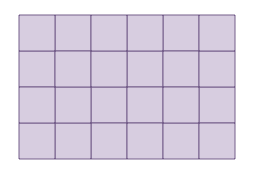Possible Answers: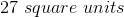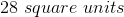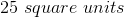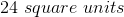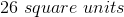Correct answer:Explanation:

The shape is made up of unit squares. We can count the number of squares within the shape to find the area.

There aresquares within the shape.

### Example Question #2 : Geometric Measurement: Understanding Concepts Of Area And Relating Area To Multiplication And To Addition

How many square units make up the area of the shape below?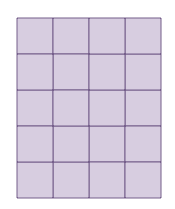Possible Answers: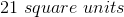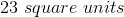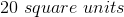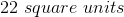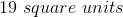Correct answer:Explanation:

The shape is made up of unit squares. We can count the number of squares within the shape to find the area.

There aresquares within the shape.

### All ISEE Lower Level Quantitative Resources